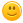# How to make a program, which a bot will quiz us?

Can we make a bot quiz us? How to scan, and reply to us is it wrong/correct?+1 vote
answered Nov 24, 2020 by (560 points)

I don't know how to make a quiz botcommented Nov 24, 2020 by (560 points)
answered Nov 25, 2020 by (78,130 points)
edited Nov 25, 2020 by Peter Minarik

# Start Simple

```#include <stdio.h>
#include <string.h>

int main()
{
char capital;
printf("What is the capital of Switzerland? : ");
scanf("%s", capital);
if (strcmp(capital, "Bern") == 0)
{
printf("Correct.\n");
}
else
{
printf("Wrong.\n");
}

return 0;
}```

# Generalise

You can make the whole process generic easily by creating an array of quiz and asking and evaluating each one of these quiz questions.
```#include <stdio.h>
#include <string.h>

#define ArrayLength(array) (sizeof(array) / sizeof(array))

typedef struct
{
const char * question;
} Quiz;

static void AskQuiz(const Quiz * quiz)
{
printf("%s : ", quiz->question);
{
printf("Correct.\n\n");
}
else
{
printf("Wrong.\n\n");
}
}

int main()
{
Quiz quiz[] =
{
{ "What is the capital of Switzerland?", "Bern" },
{ "What year did World War 2 start?", "1939" },
{ "How high is the highest mountain on Earth (in meters)?", "8848" },
};

int count = ArrayLength(quiz);

for (int i = 0; i < count; i++)
{